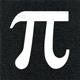# Math Is Fun Forum

Discussion about math, puzzles, games and fun.   Useful symbols: ÷ × ½ √ ∞ ≠ ≤ ≥ ≈ ⇒ ± ∈ Δ θ ∴ ∑ ∫  π  -¹ ² ³ °

You are not logged in.

## #1 2020-10-06 17:30:08

Pema
Guest

### Maths

If w is an imaginary cube root of unity and A =[ 1  0    , B= ( w 0   then
0  w].           0  1)                   (A+B) ^103 is equal to

## #2 2020-10-06 20:57:56

zetafunc
ModeratorRegistered: 2014-05-21
Posts: 2,353
Website

### Re: Maths

Hi Pema,

What do you get when you add A and B together?

What kind of matrix is it, and what do we know about powers of these matrices?

Offline

## #3 2020-10-06 22:27:02

BobRegistered: 2010-06-20
Posts: 9,228

### Re: Maths

hi Pema,

Welcome to the forum.

Thanks to zetafunc for the initial hint.

You might also find the following useful:

w^2 + w + 1 = 0  =>  w + 1 = - w^2

You can prove this equation either by substituting the complex values for each root (the working is identical) or like this:

Let w^2 + w + 1 = z ; then w^3 + w^2 + w = wz

As w^3 = 1 we end up with z = wz.  So either z = 0 or w = 1.  As we know that second isn't the case z must be 0.

Bob

Children are not defined by school ...........The Fonz
You cannot teach a man anything;  you can only help him find it within himself..........Galileo Galilei
Sometimes I deliberately make mistakes, just to test you!  …………….BobOffline